# North Middle School# Study Tips (Math)

Homework can be found on the calendar and lesson plans. Most assignments are in the math workbooks or a WRS (weekly review sheet)
Need help with homework or studying for tests? Check out the links page for direct links to videos and practice. Below you will also find helpful ideas.

Click this site to play skill based games. Pick the grade, pick the skill, practice!

Below you will find direct links to my stream videos. You must use your microsoft (school email) login to watch the videos or you can access directly in our TEAMS page under the tab "KILE MATH"

Multiplication Facts

Number Forms (word, expanded, standard)

Place Value- IXL 4th grade A.3.New
Tip: multiply by 10 (add a zero), multiply by 1/10 (take away a zero)
TRICK!!!! If they give you the answer, it's a Trick, Do the Opposite.
Example: 400 is 1/10 of what number?
400= 1/10 x _______ [ the answer is given (400), so instead of taking away a zero because we see 1/10, it's a TRICK, so we do the opposite of take one away and add a zero. Therefore, the answer is:
400= 1/10 x 4000
Value
To find the value of the underlined digit, simply write the underlined digit and turn everything else to a zero.

Standard Form- 126
Word Form- one hundred twenty-six
Expanded- 100 + 20 + 6
Expanded Extended- (1x100)+(2x10)+(6x1)
IXL- A.1, A.2, A.4, A.5

Exponents
The exponent tells the base how many times to multiply itself.
10 to the power of 5 = 10x10x10x10x10= 100,000    (a total of 5 zeros)
IXL- E.1, E.2, E.3, E.4

Multiplying multi digit numbers (Turtle Method)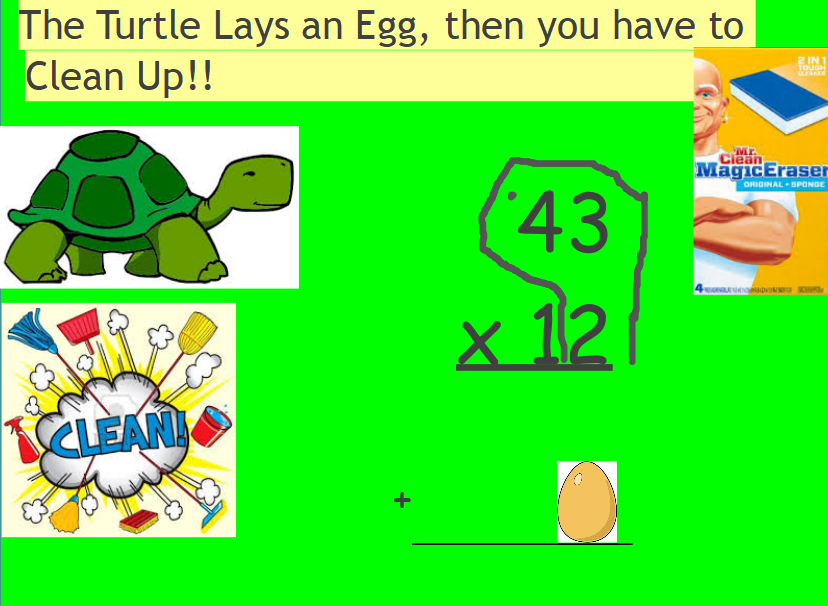Order of Operations- PEMDAS
Please Excuse My Dear Aunt Sally
1) Parentheses
2) Exponents
3) Multiply & Divide (at the same time-from left to right)
4) Add & Subtract (at the same time-from left to right)

Practice: IXL 0.5, 0.6

Expressions
Tip: put the first step in parentheses
IXL: 0.4

Chapter 1 Review Game

Below you will find direct links to my stream videos. You must use your microsoft (school email) login to watch the videos or you can access directly in our TEAMS page under the tab "KILE MATH"

Chapter 2 Long Division:

Interpret Remainder:

Chapter 2 "Times As Much"

Division Videos from Study Jams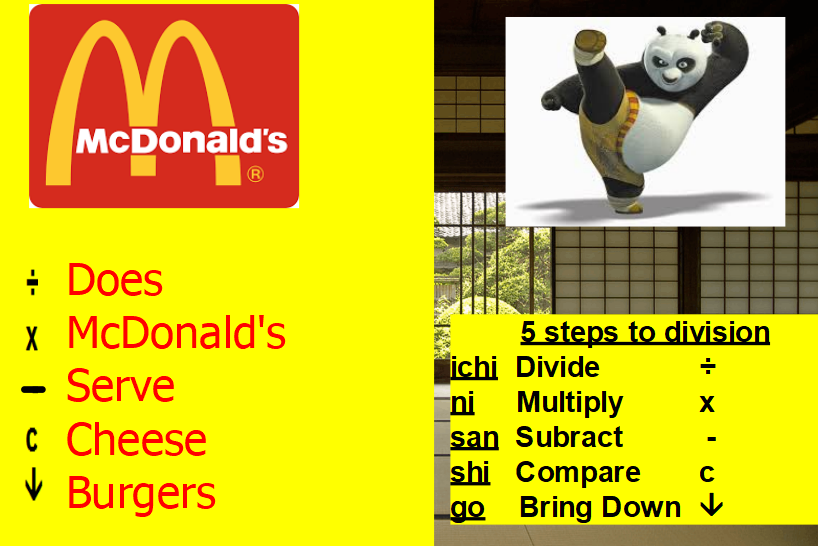Tip:
• Underline the first digit of your dividend.
• Place an "x" if your divisor does not go into your dividend. Or the number of times you can divide.
• Make sure their is a number (or "x") above every number in your dividend.

Division Word Problems
•  If you see a number followed by the word "times" (Ex. 7 times as many) Draw your boxes to find the divisor. (p.138)
• Make sure there is a WORD in your answer in a WORD problem

Interpret Remainder

NO REMAINDERS IN A WORD

Full- quotient

Need- quotient + 1

Left- remainder

How many more- Divisor - remainder

Example: There are 74 students going on a field trip. Each bus can hold 30 students.

How many buses are full?__2__ (quotient)

How many buses do they need for all of the kids to be able to go on the field trip?__3_(quotient +1)

How many students are in the bus that is not full?__14_(remainder)

How many more students are need to fill the third bus? _16__ (divisor - remainder)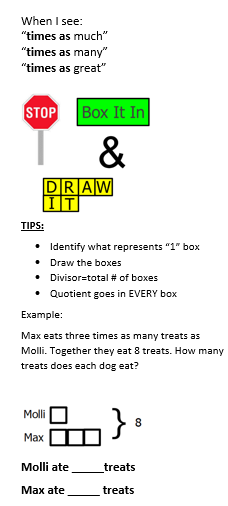Chapter 2 Review Game: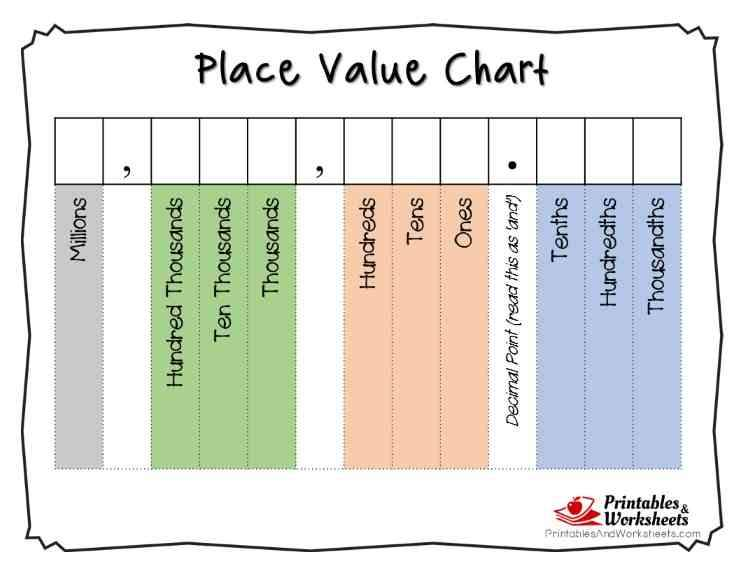Place Value Practice:IXL G.4

Comparing and Ordering Decimals

Stack ‘em- (Stack the numbers by lining up the decimals/add zeros)

Rank ‘em- (Place a number to indicate the order)

Write ‘em- (Write the numbers in order as asked in the question)

Comparing & Ordering Practice: IXL G.10, G.11

“LINE it up,                      (line up the decimals)

DROP it down,             (drop the decimal straight down to the answer)

SOLVE!”                         (solve the problem)

Tip: Once the decimal has been dropped down to the answer, forget about it and treat the problem as if it were whole numbers.

Practice: IXL H.1, H.2, H.3, H.4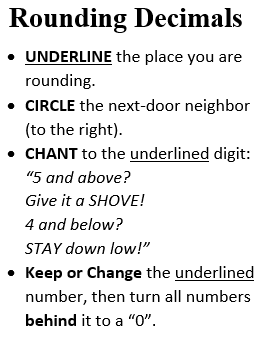Practice Rounding Decimals: IXL G.7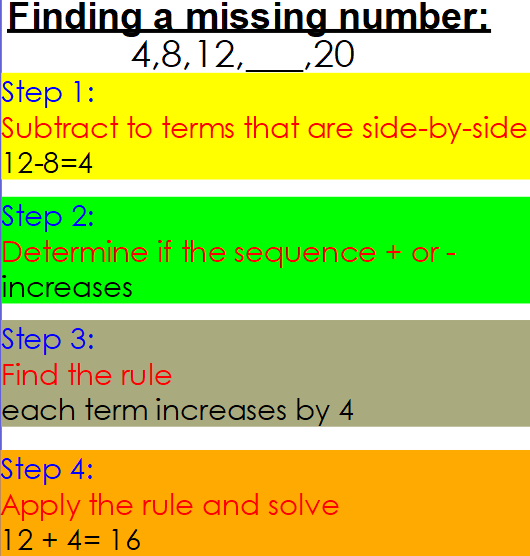Multiplying decimals

"When you multiple a decimal, move it to the right, drop it down light, move it back in site"

IXL Practice:

I.2 (multiply by powers of ten)

I.3 (multiply decimals by whole number)

I.8 (multiply two decimals)

Chapter 4 Review Game

https://jeopardylabs.com/play/multiplying-decimals-285

Dividing decimals

Move the decimal straight up to the quotient, then divide.

NO REMAINDERS!!!! Add a zero and keep going.

IXL Practice:

J.1 (divide by powers of ten)

J.3 (division with decimal quotients)

J.4 (division with decimal quotients and rounding)

https://web.microsoftstream.com/video/fb30ce59-f04a-4c3e-9bb4-2d782dddbcc7

Simplifying Fractions

https://web.microsoftstream.com/video/57976a94-e6f9-4244-a70c-caab30bb6f9c

IXL PRACTICE:

K.4 (Equivalent Fractions)

K.7 (Least Common Denominator)

K.6 (Convert mixed numbers/improper fractions)

K.5 (Lowest Terms)

L.10 (Subtract unlike denominators)

L.18 (Add mixed numbers with unlike denominators)

L.19 (Subtract mixed numbers with unlike denominators)

Regrouping Fractions When Subtracting Mixed Numbers Video:

https://quizizz.com/join?gc=015585

Multiplying Fractions

"When you multiply a fraction, multiply across, multiply across"

Multiplying with whole numbers and a fraction.
1)Put the whole number over 1
2) Multiply across (num x num/ den x den)
3) Simplify if needed.

Multiplying mixed numbers
1) Turn the mixed numbers into improper fractions
2) Multiply across
3) Simplify

IXL
M.11, M.12 (Fractions x whole number)
M.16 (Fraction of a number, word problems)
M.20 (Multiply two fractions)
M.21 (Multiply two fractions, word problems)
M.23 & M.24 (Scaling Fractions)

How to Divide Fractions: Keep, Change, Flip
1) Keep the first number/place whole number over 1
2)Change the division sign to multiplication
3) Flip the second number (ex. 3/4 would flip to be 4/3)
4) Multiply across
5) Simplify

IXL- dividing fractions (N)
N.4, N.6, N.7, N.8, N.9, N.10,

Tip: word problems. Make sure your first number is the thing you are cutting or sharing, the second number is the number of people or equal parts.

Line Plots: IXL - V.9, V.10, V.11
Tip: double check that you have counted ALL of the numbers
Average on line plot:   sum/# of x

Averages: IXL- W.2, W.6, W.10
Tip: "average" is the same as "mean"
add all the numbers to find a sum, then divide by the number of "numbers that you added"
Example: 40 + 25 + 35 + 35 + 30=165      165 divided by 5 is 33. Therefore, the average is 33
"Mode"- which number happens the MOST

Coordinate Plane: IXL- T
Tip: You must walk OVER to the elevator before going UP

Analyzing Line Graphs: IXL -T.6
Tip: Don't forget, a straight line across means NO INCREASE.

Rules and Sequences: IXL- S
Use a QR Code Reader and scan on p. 562 for a video

Converting Units of Measurement
Customary Practice- IXL- Y.1, Y.2, Y.3, Y.4, Y.5

Converting Customary Measurements

Steps to Conversions:
1) Put a 1 under known information
2) Can you convert these 2 units?(If so, move on. If not, make an extra step)
3) Place units. Cancel out matches. Circle the leftover/goal unit.
4) Put a 1 beside of the larger unit.
5) Place conversion numbers.
6) Multiply across
7) Simplify

Units of Time
IXL- X.4 (Elapsed Time)
X.5 (Start and End Time Word Problems)
X.3 (Timezones)
X.2 (Add & Subtract Mixed Time Units)
X.1 (Convert Time Units)

Metric Conversions
Metric Practice- IXL- Y.12, Y.13, Y.14, Y.15, Y.16
King Henry Doesn't Usually Drink Chocolate Milk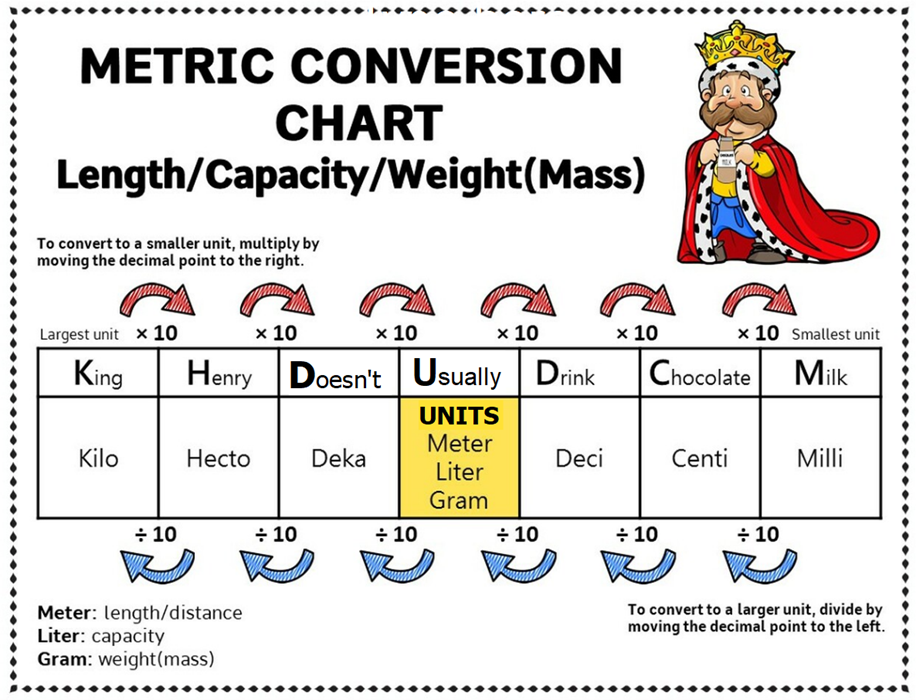Play Jeopardy Review Here:

Escape Room

Volume of Irregular Prism

IXL- Identifying Polygons
Z.1, Z.2, Z.3, Z.9, Z.10

AA.4- AA.12

Parallelograms have two sets of parallel lines,

Trapezoids only have one set at a time.

A rectangle has four right angles,

A Rhombus has four sides that are equal.

Squares have four equal sides and four right angles.

Quadrilaterals have four sides and four angles.

Parallelograms, rhombuses, trapezoids, squares, and rectangles.

And if you add the angles inside each of these,

there will be 360 degrees.

Jeopardy Review

Volume Practice
IXL-DD.13- DD.20